# Impact of Scaling on Feature Elimination with RFE¶

In this project, we will investigate how scaling the data impacts the output of a number of feature selection tools in scikit-learn.

In particular we will look into

• Linear Regression
• Decisition Tree Regression
• Support Vector Regression

For this project we will use the Auto MPG data set. Please follow the instructions in the post Working with the Auto MPG Data Set to get yourself familiar with the dataset, prepare the data for analysis and generate the auto-mpg.csv that will be used in this project.

Sections 1. in the previous post titled Impact of Scaling on Machine Learning Algorithms go over the scaling differences in the data in detail.

Below, the code that separates the data into inputs, X and output, y is provided.

In :
import pandas as pd
import numpy as np

filename = "auto-mpg.csv"
output = df.iloc[:,0]
features = df.iloc[:, 1:8]
X = features.values
y = output.values

Out:
mpg cylinders displacement horsepower weight acceleration year origin name
0 18.0 8 307.0 130.0 3504.0 12.0 70 1 chevrolet chevelle malibu
1 15.0 8 350.0 165.0 3693.0 11.5 70 1 buick skylark 320
2 18.0 8 318.0 150.0 3436.0 11.0 70 1 plymouth satellite

The y variable holds the mpg column data and the X variable holds the data in the cylinders, displacement, horsepower, weight, acceleration, year and origin columns (the name column is not used in this project).

## 2. Normalizing the Data¶

We normalize the feature data in two ways:

• rescale range to (0,1)
In :
# Rescale data (between 0 and 1)
from sklearn.preprocessing import MinMaxScaler
scaler = MinMaxScaler(feature_range=(0, 1))
rescaledX = scaler.fit_transform(X)

• standardize data so that variance is 1 and mean is zero.
In :
# Standardize data (0 mean, 1 stdev)
from sklearn.preprocessing import StandardScaler
scaler = StandardScaler().fit(X)
standardizedX = scaler.transform(X)


## 3. Test and Display Functions¶

We will use the following functions in the remaining of this post.

In :
import numpy as np
from sklearn.model_selection import KFold
from sklearn.model_selection import cross_val_score

def runModel(model, Xw, y, cv):
if cv==False:
model.fit(Xw,y)
score = model.score(Xw,y)
else:
kfold = KFold(n_splits=10, random_state=7, shuffle=True)
scores = cross_val_score(model, Xw, y, cv=kfold, scoring='r2')
score = np.array(scores).mean()
return(score)


runModel is a generic function that runs a given model and produces the r2 score with or without cross-validation.

In :
def RFAperf(ranking, modelstr, Xw, y, names, cv=False):
ranking = list(ranking)
model = eval(modelstr)
l = len(ranking)

f_inds = []
f_names = np.array([])
f_scorelist = []
for i in range(1,l+1):
f_ind = ranking.index(i)
f_inds.append(f_ind)
f_names = np.append( f_names, names[f_ind] )
Xin = Xw[:,f_inds]
score = runModel(model, Xin, y, cv)
f_scorelist.append( (f_names, score) )

return(f_scorelist)


RFAperf runs the given model using the provide feature ranking list starting with the top feature and adding the next important feature in a recursive fashion and reports the score at each step along with the features utilized in that step. RFA stands for Recursive Feature Augmentation.

In :
def rankRFE(models, Xversions, y, names):
lnames = len(names)
FAstr = 'RFE'

modelsData = []
results = pd.DataFrame([], index=range(1,lnames+1))
for inputType, Xw in Xversions:
for model in models:
modelname = str(model).partition('(')
rfe = RFE(model, 1)
# rank RFE results
rfe.fit(Xw, y)
ranking = rfe.ranking_
f_scorelist = RFAperf(ranking, str(model), Xw, y, names, cv=True)
modelsData.append( (inputType, str(model), FAstr, ranking, f_scorelist) )
f_ranking = [n for r, n in sorted( zip( ranking, names ) )]
results[modelname[0:3] + FAstr + '-' + inputType[0:2]] = f_ranking

return(modelsData, results)


rankRFE runs the RFE feature elimination algorithm on the list of models provided by the models variable to provide a feature ranking for each which then is utilized by RFAperf to produce feature augmentation performance results. The resulting data is compiled into the modelsData variable which can then be passed onto a plotting function.

In :
def plotRFAdata(modelsData, names):
n = len(modelsData)
l = len(names)

fig = plt.figure()
xvals = range(1,l+1)
colorVec = ['ro', 'go', 'bo', 'co', 'mo', 'yo', 'ko', 'rs', 'gs', 'bs', 'cs', 'ms', 'ys', 'ks']
for i in range(n):
modelData = modelsData[i]
inputType = modelData
modelstr = modelData
modelname = modelstr.partition('(')
FAstr = modelData
ranking = modelData
f_scorelist = modelData
f = np.array(f_scorelist)[:,0]
s = np.array(f_scorelist)[:,1]
labelstr = modelname[0:3] + FAstr + '-' + inputType[0:2]
plt.plot(xvals, s, colorVec[i]+'-',  label=labelstr)

fig.suptitle('Recursive Feature Augmentation Performance')
plt.ylabel('R^2')
#plt.ylim(ymax=1)
plt.xlabel('Number of Features')
plt.xlim(1-0.1,l+0.1)
plt.legend(loc='lower right', fontsize=10)
ax.set_xticks(xvals)
plt.show()


plotRFAdata extracts the information provided in the modelsData variable which is compiled by running the RFAperf function over many different models (an instance of which is the rankRFE function above utilizing the RFE method) and plots the score curve for each model/test case.

## 4. Feature Selection via RFE¶

One of the important aspects of machine learning is to determine which features are important and which features can be considered as redundant. This is especially important when the data set has hundreds or even thousands of features. This process is often referred to as feature selection.

There is a plethora of methods that is employed for feature selection (i.e., this article titled An Introduction to Variable and Feature Selection provides a nice overview, and the series of posts on this website are supplemented with Python code).

An important goal in feature selection is feature ranking. The scikit-learn package provides a versatile function called RFE to come up with a ranking of the features for a given model by recursively eliminating the most redundant feature(s). The following paragraph is from the official description of the RFE method.

Feature ranking with recursive feature elimination.

Given an external estimator that assigns weights to features (e.g., the coefficients of a linear model), the goal of recursive feature elimination (RFE) is to select features by recursively considering smaller and smaller sets of features. First, the estimator is trained on the initial set of features and weights are assigned to each one of them. Then, features whose absolute weights are the smallest are pruned from the current set features. That procedure is recursively repeated on the pruned set until the desired number of features to select is eventually reached.

The critical information regarding RFE is mentioned in its description for the estimator argument:

estimator : object

A supervised learning estimator with a fit method that updates a coef attribute that holds the fitted parameters. Important features must correspond to high absolute values in the coef array.

In other words, RFE works only if:

• There is a coef_ attribute that is provided by the model.
• Important features correspond to the high absolute values in the coef_ variable.

In this post we study the impact of scaling on how the RFE works since the coef_ attribute might directly be affected by the scaling method applied to the input feature data.

### 4.1 Linear Regression Coefficients¶

The number one model that comes to mind in regards to an output coef_ attribute is LinearRegression. In this section, we are interested in finding out how the regression coefficients are impacted by scaling.

In :
from sklearn.linear_model import LinearRegression

#names = features.columns
names = ['Cyl', 'Dis', 'Hp', 'Wt', 'Acc', 'Yr', 'Org']
lnames = len(names)
model = LinearRegression()

# Regression coefficients for unscaled, scaled and standardized input
coef_or = abs(model.fit(X,y).coef_)
coef_re = abs(model.fit(rescaledX,y).coef_)
coef_st = abs(model.fit(standardizedX,y).coef_)

#f_ranking = zip(*sorted(zip(abs(rfe.estimator_.coef_), names)))
#f_ranking = [n for r, n in sorted( zip( abs(model.coef_), names ) , reverse=True)]

import matplotlib.pyplot as plt
fig = plt.figure()
fig.suptitle('Linear Regression Coefficients (normalized abs values)')
plt.plot(range(lnames), coef_or/max(coef_or), 'ro-', label='original')
plt.plot(range(lnames), coef_re/max(coef_re), 'bo-', label='rescaled')
plt.plot(range(lnames), coef_st/max(coef_st), 'go-', label='standardized')
plt.legend(loc='upper left', fontsize=10)
plt.xlim(-0.1, 6.1)
plt.ylim(0, 1.1)
ax.set_xticks(range(lnames))
ax.set_xticklabels(names, fontsize=10)
#ax.text(0.7, 1.4, 'Ranking: %s '%f_ranking)
plt.show()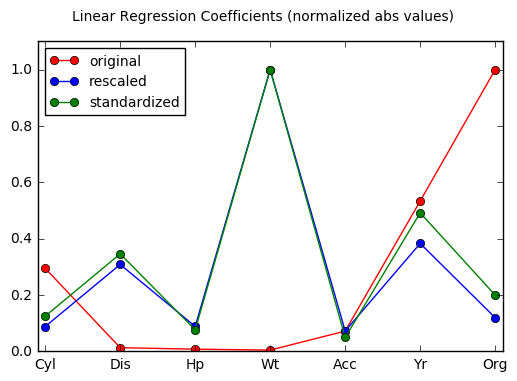What we see on the plot above is expected: when there is no scaling applied to the data the regression coefficients are adjusted such that features with large values are attenuated and vice versa. In this case, the feature importance information in the coefficients are overcome by the need to counteract scaling. Only when proper scaling is applied, the feature importance information comes about in the coefficients (as seen in the rescaled and the standardized curves). Based on the cofficients obtained after scaling the data, the top two features are Weight and Year.

### 4.2 Feature Selection using Linear Regression Coefficients¶

In this section, we would like to investigate the outcome when the regression coefficient values are utilized directly for feature ranking.

In :
from sklearn.feature_selection import RFE

model = LinearRegression()
FAstr = 'Coeffs'
#rfe = RFE(model, 1)

# prepare test
scaling = [('original', X), ('rescaled', rescaledX), ('standardized', standardizedX)]

modelsData = []
lnames = len(names)
results = pd.DataFrame([], index=range(1,lnames+1))
for inputType, Xw in scaling:

model.fit(Xw, y)

# extract ranking array from linear coefficients
ranking = np.zeros( (7,), dtype=np.int )
f_r = [n for r, n in sorted( zip( abs(model.coef_), range(lnames) ) , reverse=True)]
ranking[f_r] = range(1,lnames+1)

# get performance scores for feature accumulation based on ranking array
f_scorelist = RFAperf(ranking, str(model), Xw, y, names, cv=True)

# store all results to modelsData
modelsData.append( (inputType, str(model), FAstr, ranking, f_scorelist) )

# augment results for table display
f_ranking = [n for r, n in sorted( zip( abs(model.coef_), names ) , reverse=True)]
results[FAstr + '-' + inputType[0:2]] = f_ranking

resultsCoeffs = results

from prettypandas import PrettyPandas
from IPython.display import display
display(resultsCoeffs)
#display(PrettyPandas(resultsCoeffs))

Coeffs-or Coeffs-re Coeffs-st
1 Org Wt Wt
2 Yr Yr Yr
3 Cyl Dis Dis
4 Acc Org Org
5 Dis Hp Cyl
6 Hp Cyl Hp
7 Wt Acc Acc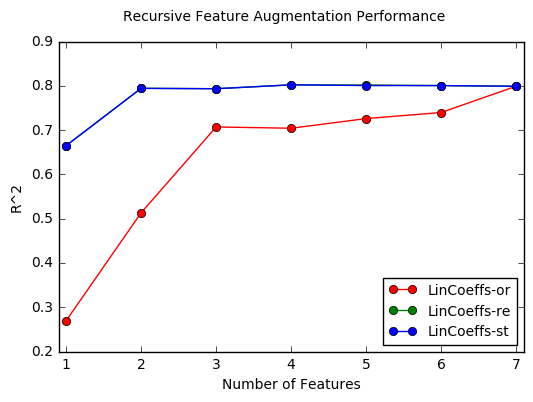We can clearly see from the table and the plot above that, when not scaled properly, the regression coefficients do not reflect the feature importance. This is obvious from the significantly inferior performance results presented by the red curve in the above plot where the feature augmentation is done according to the ranking provided in the Coeffs-or column in the table above.

One thing to note from the blue curve in the plot is how just after the two top features (Weight and Year in this case) the performance curve levels off. In other words, using just those two features is almost identical to using all.

### 4.3 RFE using Linear Regression¶

In this section we directly use the RFE method with LinearRegression as the model. The results are compared with the results of the previous section using the linear regression coefficients directly for feature ranking.

In :
models = [LinearRegression()]
names = ['Cyl', 'Dis', 'Hp', 'Wt', 'Acc', 'Yr', 'Org']
Xversions = [('original', X), ('rescaled', rescaledX), ('standardized', standardizedX)]
modelsData, results = rankRFE(models, Xversions, y, names)
display( pd.concat([resultsCoeffs, results], axis=1) )

Coeffs-or Coeffs-re Coeffs-st LinRFE-or LinRFE-re LinRFE-st
1 Org Wt Wt Cyl Wt Wt
2 Yr Yr Yr Org Yr Yr
3 Cyl Dis Dis Yr Dis Org
4 Acc Org Org Acc Hp Dis
5 Dis Hp Cyl Hp Org Hp
6 Hp Cyl Hp Dis Cyl Cyl
7 Wt Acc Acc Wt Acc Acc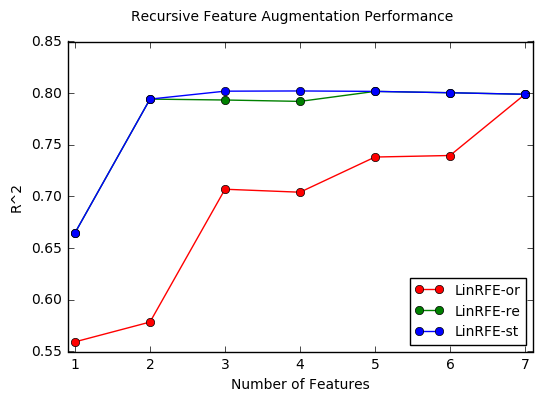It is clear from this plot that proper scaling is crucial for the RFE method to work with LinearRegression as desired. This is in stark contrast to the LinearRegression model's performance immunity against scaling.

One thing to note from the table above is the difference between the rankings chosen by RFE and directly from the regression coefficients. Although still inferior, the RFE feature rankings seems to be much better than what the regression coefficients indicate. The inner workings of the RFE function must involve other steps than just relying on the coefficents themselves (which is beyond the scope of this study).

### 4.4 RFE with Linear and Ridge Regression¶

In this section we compare the RFE method using Linear and Ridge regression.

In :
from sklearn.linear_model import Ridge

Lmodels = [LinearRegression(), Ridge()]
names = ['Cyl', 'Dis', 'Hp', 'Wt', 'Acc', 'Yr', 'Org']
Xversions = [('original', X), ('rescaled', rescaledX), ('standardized', standardizedX)]
modelsData, results = rankRFE(Lmodels, Xversions, y, names)
display(results)

LinRFE-or RidRFE-or LinRFE-re RidRFE-re LinRFE-st RidRFE-st
1 Cyl Cyl Wt Wt Wt Wt
2 Org Org Yr Yr Yr Yr
3 Yr Yr Dis Hp Org Org
4 Acc Acc Hp Org Dis Dis
5 Hp Hp Org Cyl Hp Hp
6 Dis Dis Cyl Dis Cyl Cyl
7 Wt Wt Acc Acc Acc Acc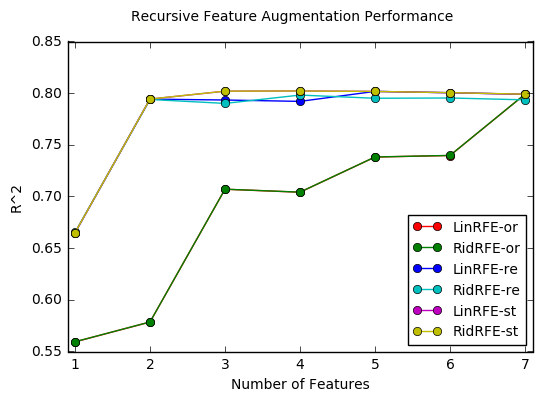The results above show that LinearRegression and Ridge models perform very similarly when used with RFEexcept the case of rescaling to the (0,1) range.

### 4.5 RFE with Ridge, Lasso and ElasticNet¶

In this section we compare the RFE method using Linear, Lasso and ElasticNet regression.

In :
from sklearn.linear_model import Lasso
from sklearn.linear_model import ElasticNet

Lmodels = [LinearRegression(), Lasso(), ElasticNet()]
names = ['Cyl', 'Dis', 'Hp', 'Wt', 'Acc', 'Yr', 'Org']
Xversions = [('original', X), ('rescaled', rescaledX), ('standardized', standardizedX)]
modelsData, results = rankRFE(Lmodels, Xversions, y, names)
display(results)
plt.rcParams["figure.figsize"] = [8, 6]
plt.rcParams["figure.figsize"] = [6, 4]

LinRFE-or LasRFE-or ElaRFE-or LinRFE-re LasRFE-re ElaRFE-re LinRFE-st LasRFE-st ElaRFE-st
1 Cyl Yr Yr Wt Cyl Cyl Wt Wt Wt
2 Org Wt Wt Yr Yr Dis Yr Yr Dis
3 Yr Hp Hp Dis Org Org Org Org Yr
4 Acc Org Org Hp Acc Wt Dis Hp Hp
5 Hp Acc Dis Org Wt Yr Hp Acc Cyl
6 Dis Dis Acc Cyl Hp Hp Cyl Dis Org
7 Wt Cyl Cyl Acc Dis Acc Acc Cyl Acc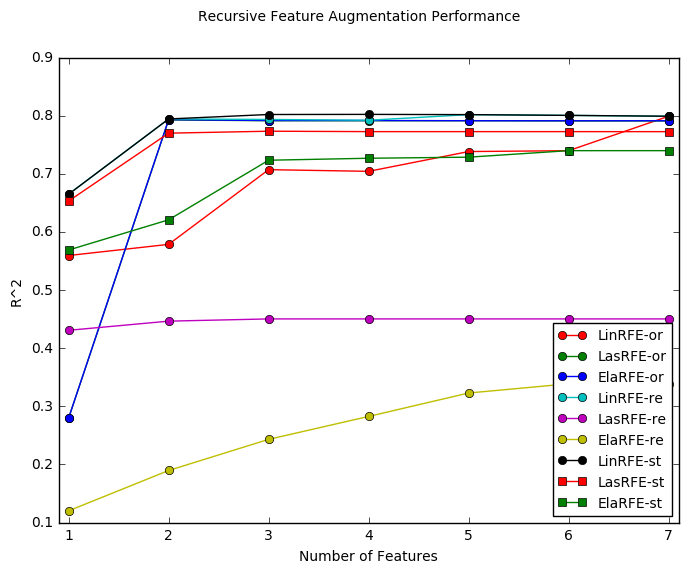A number of interesting things are happening above:

• RFE with Lasso and ElasticNet regression are not impacted significantly (except in their choice for the top feature) for not being scaled. This is in significant contrast to Linear and Ridge regression methods.
• However, both Lasso and ElasticNet perform terribly when the inputs are scaled to the (0, 1) range.
• Lasso regression works best with RFE when inputs are standardized. There is, however, a slight degradation in overall performance compared to the unscaled case.
• ElasticNet is much worse than Lasso with standardized data. There is, however, a significant degradation in overall performance compared to the unscaled case.

### 4.6 RFE with Support Vector Regression¶

In this section we study feature ranking with the RFE method using Support Vector Regression.

In :
from sklearn.svm import SVR

Smodels = [SVR(kernel="linear")]
Xversions = [('original', X), ('rescaled', rescaledX), ('standardized', standardizedX)]
names = ['Cyl', 'Dis', 'Hp', 'Wt', 'Acc', 'Yr', 'Org']
modelsData, results = rankRFE(Smodels, Xversions, y, names)

In :
display(results)

SVRRFE-or SVRRFE-re SVRRFE-st
1 Cyl Wt Wt
2 Org Yr Yr
3 Yr Cyl Org
4 Acc Org Cyl
5 Hp Hp Hp
6 Dis Dis Acc
7 Wt Acc Dis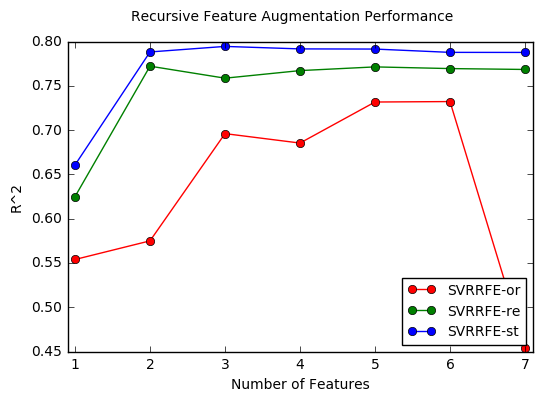RFE with Support Vector Regression (SVR) is greatly impacted by proper scaling as expected. There is a clear advantage in using the standardized version of the input.

Also note that RFE only works with SVR when the kernel is chosen to be linear. It does not work with the nonlinear kernels. For example with SVR(kernel='rbf') you get the following error:

RuntimeError: The classifier does not expose coef_ or feature_importances_ attributes

### 4.7 RFE with K-nearest Neighbor Regression¶

The KNN method does not provide any metric for feature importance and therefore cannot be used with RFE.

### 4.8 RFE with Decision Tree Regression¶

In this section we study feature ranking with RFE using Decision Tree Regression.

In :
from sklearn.tree import DecisionTreeRegressor

Dmodels = [DecisionTreeRegressor()]
names = ['Cyl', 'Dis', 'Hp', 'Wt', 'Acc', 'Yr', 'Org']
Xversions = [('original', X), ('rescaled', rescaledX), ('standardized', standardizedX)]
modelsData, results = rankRFE(Dmodels, Xversions, y, names)
display(results)

DecRFE-or DecRFE-re DecRFE-st
1 Dis Dis Dis
2 Wt Wt Wt
3 Yr Yr Yr
4 Hp Hp Hp
5 Acc Acc Acc
6 Org Org Org
7 Cyl Cyl Cyl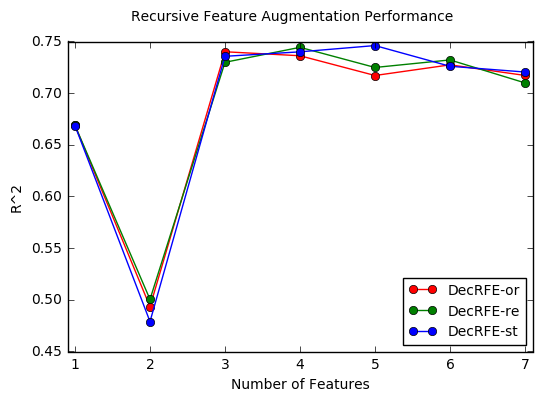As expected, we do not see any significant difference in using the RFE with Decision Tree Regression in regards to scaling. However, the resulting feature ranking is somewhat different; RFE selects Displacement as the top ranking feature (as opposed to the Weight feature chosen with all the other models).

Also, there seems to be problem when running with both the Displacement and Weight features which goes back to normal when Year is added as the third feature.

### 4.9 RFE with Bagging Algorithms¶

None of the Bagging Algorithms in scikit-learn is supported with RFE:

• ExtraTreesRegressor
• RandomForestRegressor (although this link discusses a way to circumvent this problem for SVC)
• BaggingRegressor

### 4.10 RFE with Boosting Algorithms¶

In this section we study feature ranking with RFE using Boosting Algorithms.

In :
from sklearn.ensemble import AdaBoostRegressor

num_trees = 100
seed = 7
names = ['Cyl', 'Dis', 'Hp', 'Wt', 'Acc', 'Yr', 'Org']
Xversions = [('original', X), ('rescaled', rescaledX), ('standardized', standardizedX)]
modelsData, results = rankRFE(BSTmodels, Xversions, y, names)
display(results)

1 Wt Dis Wt Dis Wt Dis
2 Yr Acc Yr Acc Yr Acc
3 Dis Wt Hp Wt Hp Wt
4 Hp Hp Dis Hp Dis Hp
5 Acc Yr Acc Yr Acc Yr
6 Org Cyl Cyl Cyl Cyl Cyl
7 Cyl Org Org Org Org Org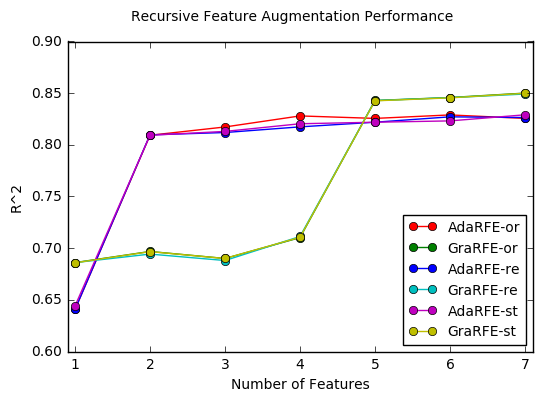As expected, we do not see any significant difference in using the RFE with Boosting Algorithms (which are all decision-tree based) in regards to scaling.

The AdaBoost algorithm behavior is very similar to previous models in terms of the performance trends observed during feature augmentation. Similar to the DecisionTreeRegressor but in contrast to the other models, the Origin feature is close to the bottom of the ranking list.

The GradientBoosting algorithm, on the other hand, prefers a feature ranking quite different than all other models. Similar to the the DecisionTreeRegressor, the Displacement feature is ranked at the top but the favorite Weight and Year features come at the 3rd and the 5th rankings. Also note that the regression performance does not start leveling off until the 5th feature is selected which is in great contrast to other models which only require 2 features to reach this level.

## 5. Comparison of RFE using standardized inputs¶

In this section we compare all the compatible models with RFE using only the standardized inputs which seems to be the desired scaling method considering all the results above.

In :
Lmodels = [LinearRegression(), Lasso(), ElasticNet()] # Ridge is similar to Linear, therefore excluded
Smodels = [SVR(kernel="linear")]
Dmodels = [DecisionTreeRegressor()]

Allmodels = Lmodels + Smodels + Dmodels + BSTmodels
names = ['Cyl', 'Dis', 'Hp', 'Wt', 'Acc', 'Yr', 'Org']
Xversions = [('standardized', standardizedX)]
modelsData, results = rankRFE(Allmodels, Xversions, y, names)
display(results)

LinRFE-st LasRFE-st ElaRFE-st SVRRFE-st DecRFE-st AdaRFE-st GraRFE-st
1 Wt Wt Wt Wt Dis Wt Dis
2 Yr Yr Dis Yr Wt Yr Acc
3 Org Org Yr Org Yr Hp Wt
4 Dis Hp Hp Cyl Hp Dis Hp
5 Hp Acc Cyl Hp Acc Acc Yr
6 Cyl Dis Org Acc Org Cyl Cyl
7 Acc Cyl Acc Dis Cyl Org Org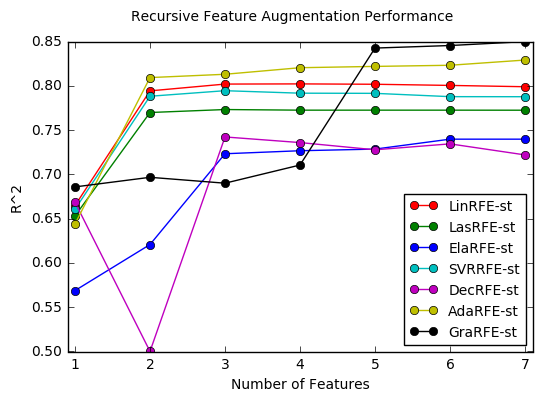Below we will classify the models based on the minimum number of features that can be selected as indicated by the plot above:

• Models with 2 features selectable:
• Linear, Lasso, Support Vector and AdaBoost regressors:
• Features: Weight and Year
• Models with 3 features selectable:
• DecisionTree and ElasticNet regressors:
• Features: Displacement, Weight and Year
• Models with 5 features selectable:
• GradientBoosting regressor:
• Features: Displacement, Acceleration, Weight, Horsepower and Year

## 6. Using RFECV - RFE with cross validation¶

The RFECV method provides the additional capability to include cross-validation in the feature elimination process.

In :
from sklearn.feature_selection import RFECV

Xw = standardizedX
Allmodels = Lmodels + Smodels + Dmodels + BSTmodels
print(names)

l = len(names)
plt.figure()
xvals = range(1,l+1)
colorVec = ['ro', 'gs', 'bo', 'cs', 'mo', 'ys', 'ko']
i = 0
for model in Allmodels:

#rfecv = RFECV(estimator=model, cv=10, scoring='neg_mean_squared_error')
rfecv = RFECV(estimator=model, cv=10, scoring='r2')
rfecv.fit(Xw, y)

modelname = str(model).partition('(')
print("Model: %s") % modelname
print("Num Features: %d") % rfecv.n_features_
#print("Selected Features: %s") % rfecv.support_
print("Feature Ranking: %s") % rfecv.ranking_

# Plot number of features VS. cross-validation scores
labelstr = modelname[0:3] + '-st'
plt.plot(xvals, rfecv.grid_scores_, colorVec[i]+'-', label=labelstr)
i = i+1

plt.title('RFECV: RFE with cross validation')
plt.xlabel("Number of features selected")
plt.ylabel("Cross validation score")
plt.legend(loc='lower right', fontsize=10)
plt.show()

['Cyl', 'Dis', 'Hp', 'Wt', 'Acc', 'Yr', 'Org']
Model: LinearRegression
Num Features: 6
Feature Ranking: [1 1 1 1 2 1 1]
Model: Lasso
Num Features: 3
Feature Ranking: [5 4 2 1 3 1 1]
Model: ElasticNet
Num Features: 7
Feature Ranking: [1 1 1 1 1 1 1]
Model: SVR
Num Features: 3
Feature Ranking: [2 5 3 1 4 1 1]
Model: DecisionTreeRegressor
Num Features: 5
Feature Ranking: [3 1 1 1 1 1 2]
Num Features: 6
Feature Ranking: [1 1 1 1 1 1 2]
Num Features: 7
Feature Ranking: [1 1 1 1 1 1 1]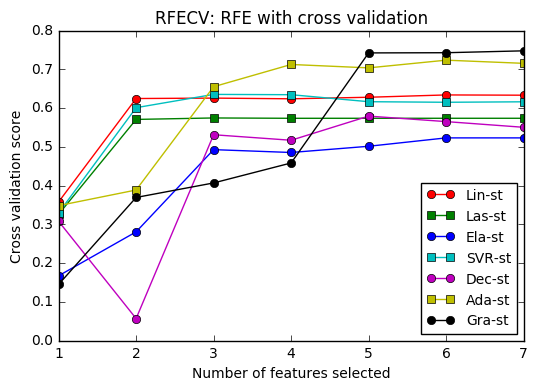The curves in this plot is very similar to those in the plot obtained in the previous section (Section 5). Both methods use 10-fold cross-validation. The RFECV function, unfortunately, does not explicitly put out the full feature ranking information therefore the top features selected is not included below.

• Models with 2 features selectable:
• Linear, Lasso, Support Vector regressors
• Models with 3 features selectable:
• ElasticNet regressor
• Models with 4 features selectable:
• AdaBoost regressor
• Models with 5 features selectable:
• Decision Tree and GradientBoosting regressors

The performance results reported in the above plot is much worse than the one provided in Section 5.

The reporting capability provided by RFECV is somewhat limited:

• RFECV automatically determines the number of features selected based on the peak performance point in the above plot. This is clearly observed in the case of the DecisionTreeRegressor as the number of features selected is 5. There is no way to change this criteria.
• Once the number of features are selected, the ranking information within that set is not reported. Therefore, most of the time, the full feature ranking is not available.
• There is no way to set the desired number of features (such as in RFE) or disable automatic selection.
• The number of features selected is strongly dependent on the number of folds set for cross-validation.

## 7. Selecting the top features¶

Although the top features ranked by RFE using different models vary, the Weight, Year, Displacement and Origin features seem to rank high across almost all models. Below we compare the performance difference between selecting all the models vs selecting top performing combination of these features.

In :
Xw = standardizedX
names = ['Cyl', 'Dis', 'Hp', 'Wt', 'Acc', 'Yr', 'Org']
selections = [ ['Wt', 'Yr'],
['Wt', 'Yr', 'Org'],
['Wt', 'Yr', 'Dis'] ]
Allmodels = Lmodels + Smodels + Dmodels + BSTmodels

plt.rcParams["figure.figsize"] = [7.5, 5]
fig = plt.figure()
colorVec = ['gs', 'bo', 'cs', 'mo', 'ys', 'ko']
for i, selection in enumerate(selections):
top_inds = [ names.index(a) for a in selection ]
Xin = Xw[:, top_inds]

scores = []
modelnames = []
for model in Allmodels:
modelname = str(model).partition('(')
modelname = modelname.partition('R')
modelnames.append(modelname)
score = runModel(model, Xin, y, cv=True)
scores.append(score)
plt.plot(scores, colorVec[i] + '-', label=','.join(selection))

scores = []
for model in Allmodels:
score = runModel(model, Xw, y, cv=True)
scores.append(score)
plt.plot(scores, 'ro-', label='All')

fig.suptitle('Feature Selection Performance')
ax.set_xticks(range(7))
ax.set_xticklabels(modelnames, fontsize=10, rotation=60)
plt.xlim(-0.1, 6.1)
plt.ylabel("R^2")
plt.legend(loc='upper left', fontsize=11)
plt.show()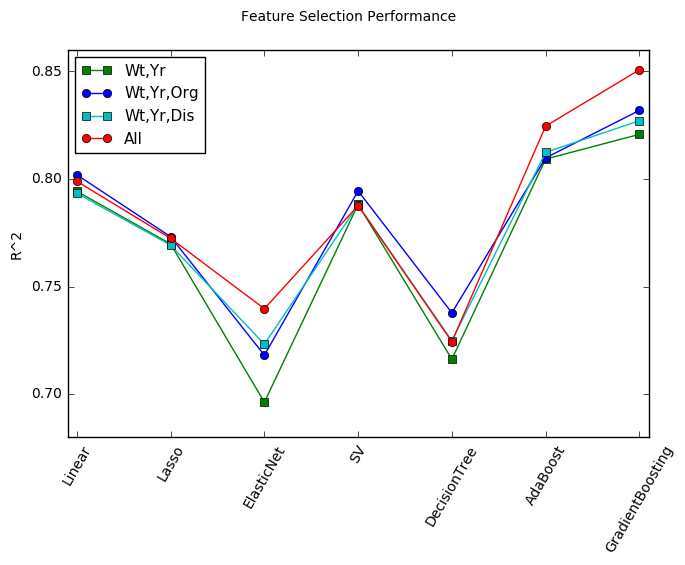As seen from the plot above:

• Linear, Lasso and SupportVector models suffer very little performance loss when using just Weight and Year, however perform superior when Origin is added as a third feature.
• AdaBoost, GradientBoosting and ElasticNet suffer about 3-5% performance loss when features are removed.
• DecisionTree performs even better when redundant features are removed. SupportVector model exhibits a small increase in performance also with less features.

## 8. Summary¶

The following models are sensitive to scaling when used with RFE:

• LinearRegression, Ridge and SVR: unscaled does not work
• Lasso and ElasticNet: rescaled does not work

DecisionTreeRegressor, AdaBoostRegressor and GradientBoostingRegressor models are not affected by scaling when used in RFE.

RFECV is not very useful due to lack of outputs.

We have also briefly looked at the impact of feature selection on performance in the last section and have observed slight loss or even increase in performance. Note, however, that the models used were not optimized and were called with default parameters.

In [ ]: